Definite Integral as a limit of a sum

Chapter 7 Class 12 Integrals
Concept wise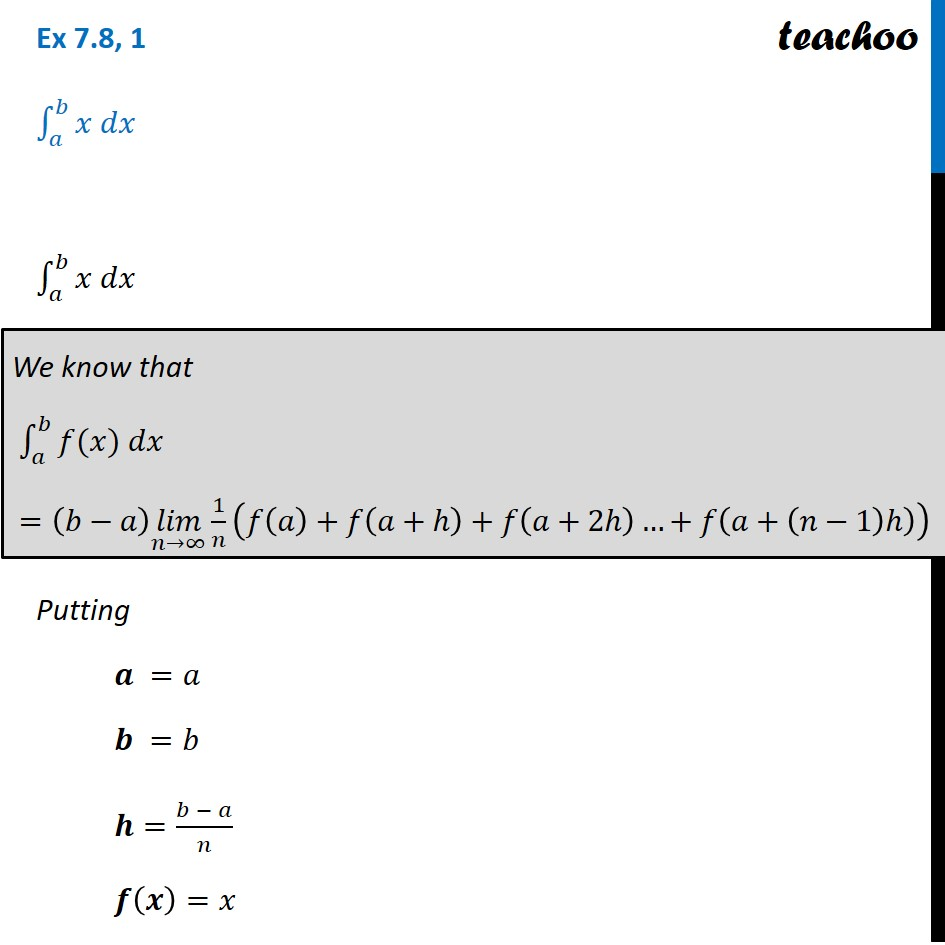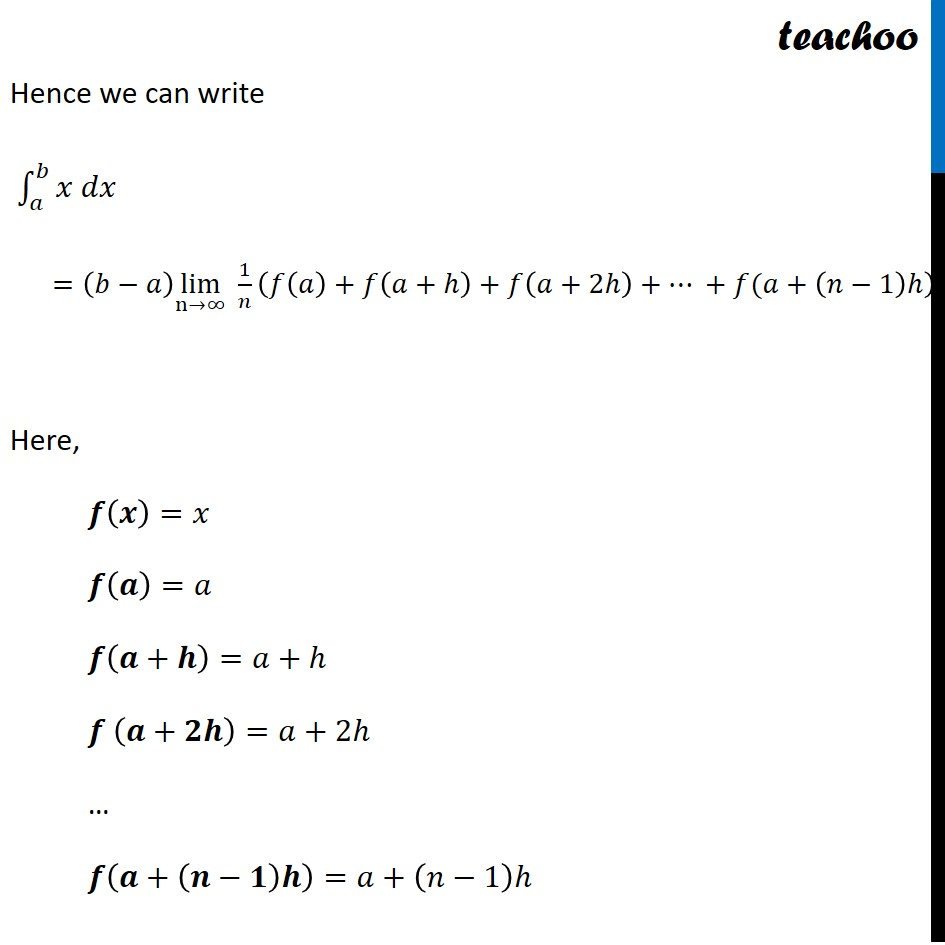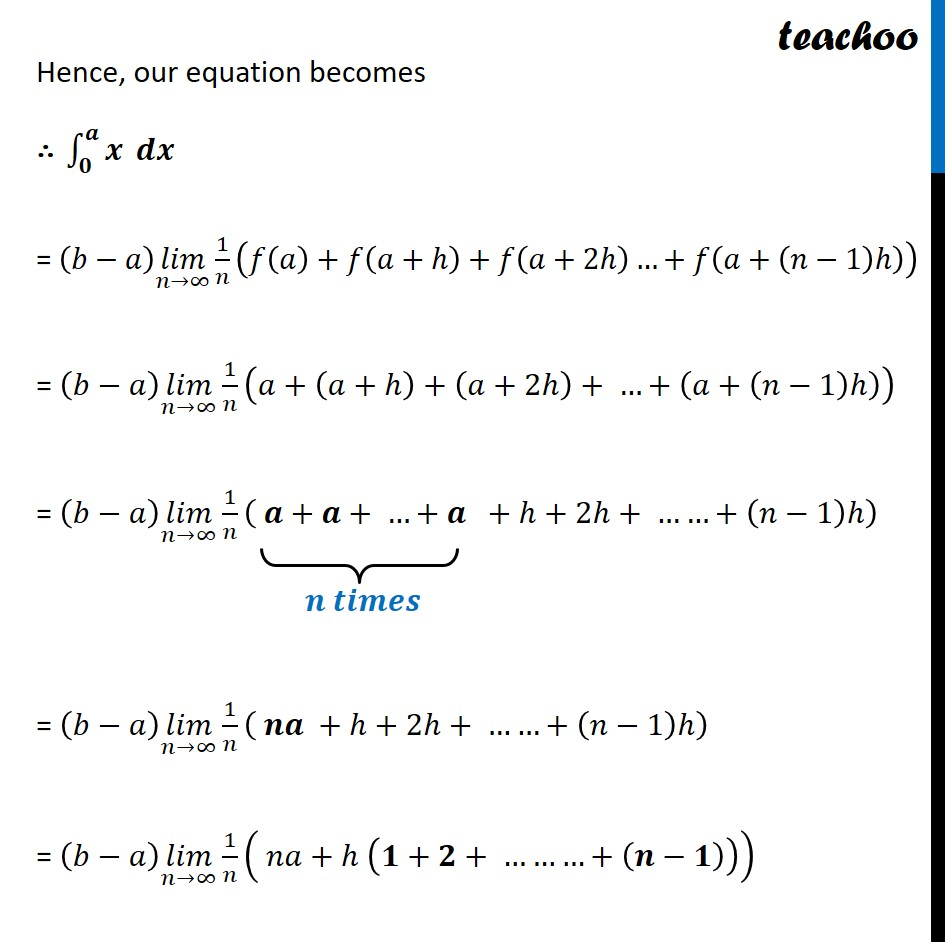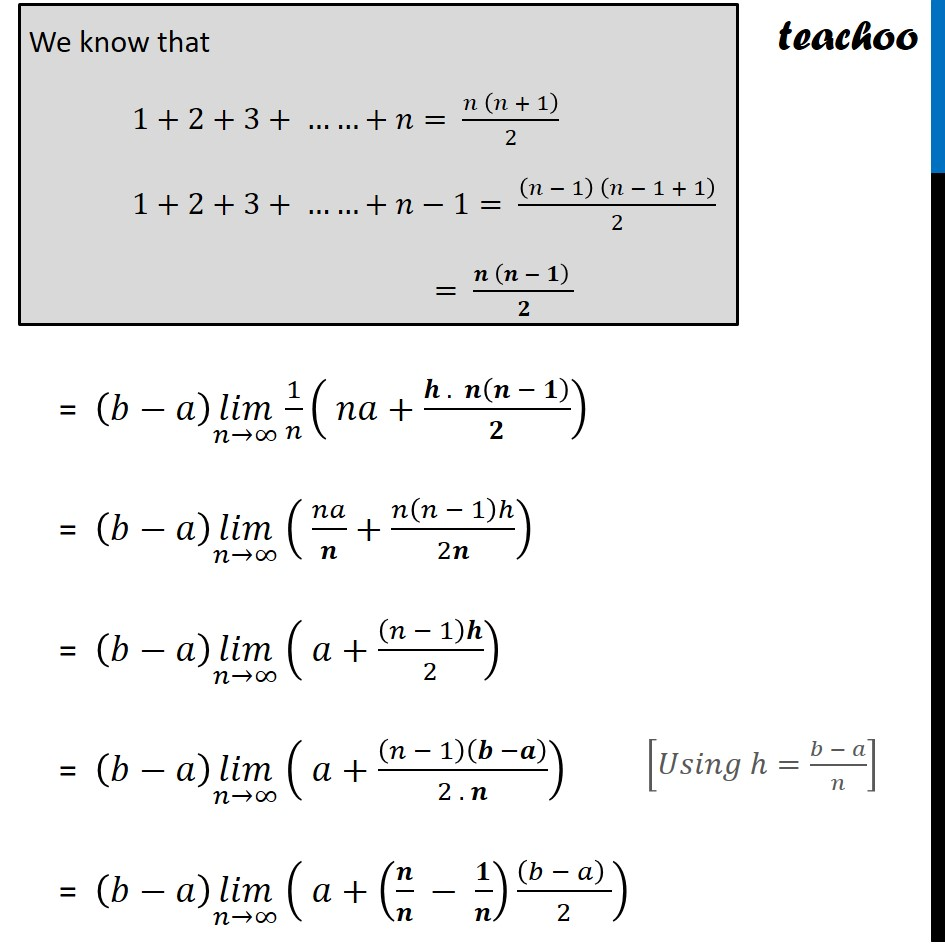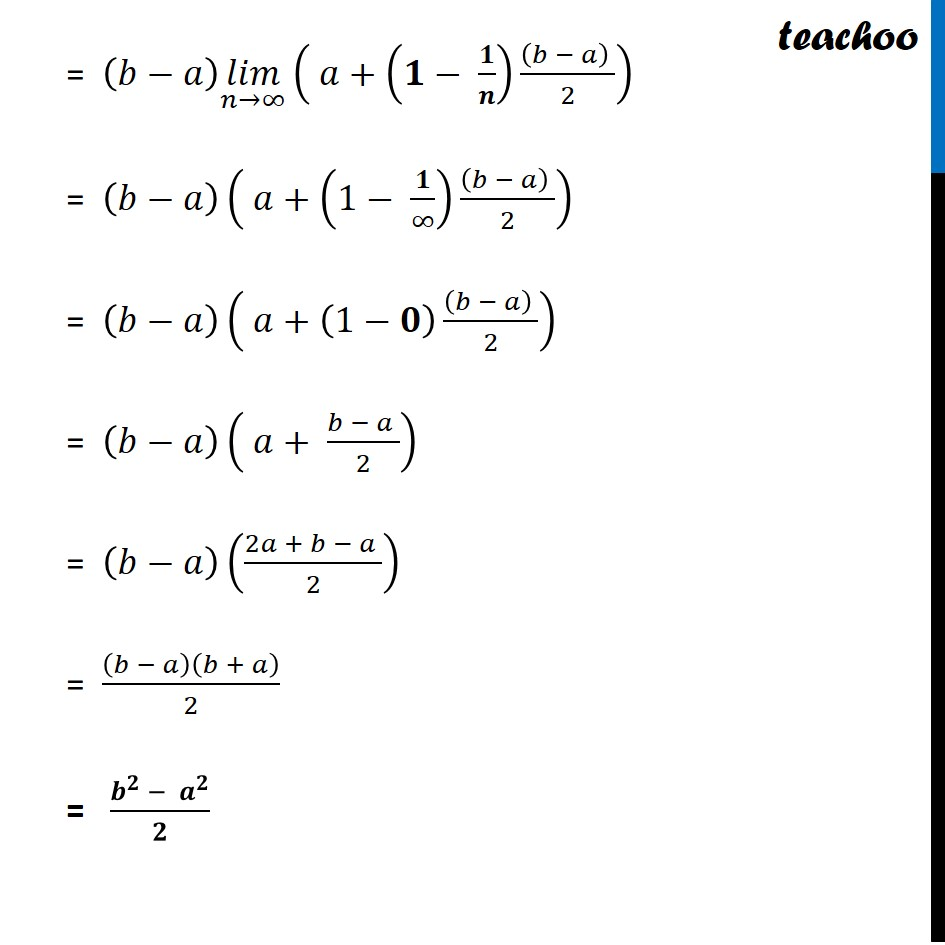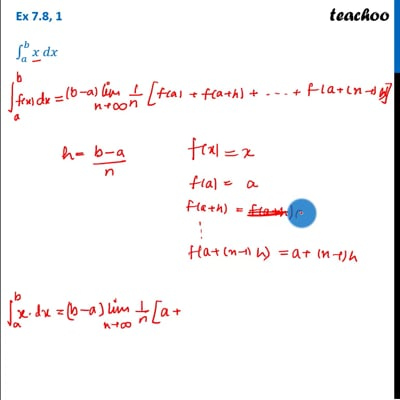This video is only available for Teachoo black users

Solve all your doubts with Teachoo Black (new monthly pack available now!)

### Transcript

Ex 7.8, 1 ∫1_𝑎^𝑏▒〖𝑥 𝑑𝑥〗 ∫1_𝑎^𝑏▒〖𝑥 𝑑𝑥〗 Putting 𝒂 =𝑎 𝒃 =𝑏 𝒉=(𝑏 − 𝑎)/𝑛 𝒇(𝒙)=𝑥 We know that ∫1_𝑎^𝑏▒〖𝑓(𝑥) 𝑑𝑥〗 =(𝑏−𝑎) (𝑙𝑖𝑚)┬(𝑛→∞) 1/𝑛 (𝑓(𝑎)+𝑓(𝑎+ℎ)+𝑓(𝑎+2ℎ)…+𝑓(𝑎+(𝑛−1)ℎ)) Hence we can write ∫1_𝑎^𝑏▒〖𝑥 𝑑𝑥〗 =(𝑏−𝑎) lim┬(n→∞) 1/𝑛 (𝑓(𝑎)+𝑓(𝑎+ℎ)+𝑓(𝑎+2ℎ)+… +𝑓(𝑎+(𝑛−1)ℎ) Here, 𝒇(𝒙)=𝑥 𝒇(𝒂)=𝑎 𝒇(𝒂+𝒉)=𝑎+ℎ 𝒇 (𝒂+𝟐𝒉)=𝑎+2ℎ … 𝒇(𝒂+(𝒏−𝟏)𝒉)=𝑎+(𝑛−1)ℎ Hence, our equation becomes ∴ ∫_𝟎^𝒂▒𝒙 𝒅𝒙 = (𝑏−𝑎) (𝑙𝑖𝑚)┬(𝑛→∞) 1/𝑛 (𝑓(𝑎)+𝑓(𝑎+ℎ)+𝑓(𝑎+2ℎ)…+𝑓(𝑎+(𝑛−1)ℎ)) = (𝑏−𝑎) (𝑙𝑖𝑚)┬(𝑛→∞) 1/𝑛 (𝑎+(𝑎+ℎ)+(𝑎+2ℎ)+ …+(𝑎+(𝑛−1)ℎ)) = (𝑏−𝑎) (𝑙𝑖𝑚)┬(𝑛→∞) 1/𝑛 ( 𝒂+𝒂+ …+𝒂 +ℎ+2ℎ+ ……+(𝑛−1)ℎ) = (𝑏−𝑎) (𝑙𝑖𝑚)┬(𝑛→∞) 1/𝑛 ( 𝒏𝒂 +ℎ+2ℎ+ ……+(𝑛−1)ℎ) = (𝑏−𝑎) (𝑙𝑖𝑚)┬(𝑛→∞) 1/𝑛 ( 𝑛𝑎+ℎ (𝟏+𝟐+ ………+(𝒏−𝟏))) 𝒏 𝒕𝒊𝒎𝒆𝒔 We know that 1+2+3+ ……+𝑛= (𝑛 (𝑛 + 1))/2 1+2+3+ ……+𝑛−1= ((𝑛 − 1) (𝑛 − 1 + 1))/2 = (𝒏 (𝒏 − 𝟏) )/𝟐 = (𝑏−𝑎) (𝑙𝑖𝑚)┬(𝑛→∞) 1/𝑛 ( 𝑛𝑎+(𝒉 . 𝒏(𝒏 − 𝟏))/𝟐) = (𝑏−𝑎) (𝑙𝑖𝑚)┬(𝑛→∞) ( 𝑛𝑎/𝒏+𝑛(𝑛 − 1)ℎ/2𝒏) = (𝑏−𝑎) (𝑙𝑖𝑚)┬(𝑛→∞) ( 𝑎+(𝑛 − 1)𝒉/2) = (𝑏−𝑎) (𝑙𝑖𝑚)┬(𝑛→∞) ( 𝑎+(𝑛 − 1)(𝒃 −𝒂)/(2 . 𝒏)) = (𝑏−𝑎) (𝑙𝑖𝑚)┬(𝑛→∞) ( 𝑎+(𝒏/𝒏 − 𝟏/𝒏) ((𝑏 − 𝑎) )/2) [𝑈𝑠𝑖𝑛𝑔 ℎ=(𝑏 − 𝑎)/𝑛] = (𝑏−𝑎) (𝑙𝑖𝑚)┬(𝑛→∞) ( 𝑎+(𝟏− 𝟏/𝒏) ((𝑏 − 𝑎) )/2) = (𝑏−𝑎)( 𝑎+(1− 𝟏/∞) ((𝑏 − 𝑎) )/2) = (𝑏−𝑎)( 𝑎+(1−𝟎) ((𝑏 − 𝑎) )/2) = (𝑏−𝑎)( 𝑎+ (𝑏 − 𝑎 )/2) = (𝑏−𝑎)((2𝑎 + 𝑏 − 𝑎 )/2) = (𝑏 − 𝑎)(𝑏 + 𝑎)/2 = (𝒃^𝟐 − 𝒂^𝟐)/𝟐